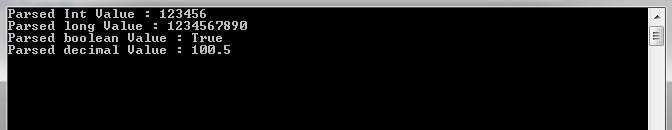“What is the difference between Int32.TryParse() & Int32.Parse() ? Or When to use TryParse() and Parse() ? ” Or Difference between Decimal.TryParse() & Decimal.Parse() ? These type of questions you might expect in C#.Net interviews. Lets explore it to understand the usage and key difference between TryParse() and Parse() methods. Before going in detail, if you haven’t gone through my article on Datatypes in C#, then please do it here

## Parse() :

All numeric primitive types (int, decimal, float, long , bool etc) have a static method as Parse() . This method takes string value as parameter and tries to convert to corresponding numeric type.

Syntax and usage :  We can access the method using dot (.) operator as follows.

The method takes a string value as an input parameter and returns the resultant converted value. Lets take an example to see its usage.

In below sample code, we are trying to convert  string value "123456"  to int type , string value "1234567890"  to long type, string value "true"  to booloean type and string value "100.5"  to decimal type using Parse method.

When above code runs then it successfully converts the string variables to their corresponding type as shown below in the quick watch window.

Output:In the above example, the CLR successfully converted the string values to their corresponding type. What would happen, if there is invalid string or if the conversion fails. Lets looks at that aspect as well.

In below example, we are trying to convert a string value "Hello"  to int type using Parse method.

When above code runs then it throws a System.FormatException as Input string was not in a correct format. , which is valid case, because string type "Hello"  cannot be parsed to type int .

Above image displays the exception details and its clear that if there is no conversion then Parse() method throws a System.FormatException .

So we can say that , Parse() method tries to convert string variable to a corresponding primitive (int, decimal, float, long , bool etc) data type. It will throws an exception when the conversion fails.

#### Where to use Parse() ? :

• In scenarios, where you expect valid string type that is convertible to specified primitive type.
• While using Parse(), Proper try catch block should be implemented to catch if any exception occurs during conversion.

## TryParse() :

All numeric primitive data types (int, decimal, float, long , bool etc) also have a static method as TryParse() . It is similar to Parse() and used to convert string type to specified numeric type, however if there is a conversion failure, then it returns converted value as 0 and instead of throwing exception, it returns a boolean false value.

Syntax and usage : It is a static method and is accessible through dot (.) operator as follows.

The method takes a string value as an input parameter, the resultant conversion value as out parameter, a return value of True for successful conversion and False for unsuccessful conversion.

In below sample code, we are trying to convert string value "001234"  to int using TryParse method.

When above code runs then it successfully converts the input string and returns True, the resultant converted value is assigned to the out parameter as displayed in the below quick watch window.

Output :In the above example, the CLR successfully converted the string values to their corresponding type and returns a boolean value as True if conversion successful and False if conversion failed.

In below example, we pass a string variable as "Hello World"  and try to convert it to int type using TryParse()  method.

When above code runs then it returns False value from TryParse() method, as it fails to convert "Hello World" to type int and if you check the variable number then it has assigned a 0 value.

One thing to note here is that, TryParse method doesn’t throw any error in case of conversion failure.